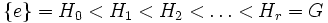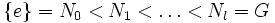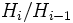# Finite composition length implies every subnormal series can be refined to a composition series

## Statement

If$G$ is a Group of finite composition length (?), and we have a subnormal series of$G$ of the form:$\{ e \} = H_0 < H_1 < H_2 < \dots < H_r = G$

where each$H_i$ is a proper normal subgroup of$H_{i+1}$, then there exists a composition series:$\{ e \} = N_0 < N_1 < \dots < N_l = G$

where each$H_i$ occurs as$N_j$ for some$j$.

(Note that if the original subnormal series has repetitions, we can throw out the repetitions, and even if it does not start at the trivial group, we can insert the trivial group. Thus, there is no loss of generality in assuming that the subnormal series is of the above form).

## Proof

### Proof idea

The idea is to find a composition series of$H_i/H_{i-1}$ for each$i$ and use the lattice isomorphism theorem to fill in subgroups between$H_{i-1}$ and$H_i$ that form part of a composition series for$G$. Thus, the main question is: does$H_i/H_{i-1}$ have a composition series?

### Hands-off proof

Given: Group$G$ of finite composition length with a strictly ascending subnormal series$H_i$.

To prove:$H_i$ can be refined to a composition series.

Proof:

1. By facts (1) and (2), each quotient$H_i/H_{i-1}$ has finite composition length. The lattice isomorphism theorem (Fact (3)) allows us to therefore get a series of subgroups from$H_{i-1}$ to$H_i$ with the factor groups being simple.
2. Putting together all these series provides a composition series for$G$ that refines the$H_i$s.

### Hands-on proof

Given: Group$G$ of finite composition length with a strictly ascending subnormal series$H_i$.

To prove:$H_i$ can be refined to a composition series.

Proof: The idea is to use the fact that$G$ has some composition series of finite length to construct a composition series of finite length that refines the$H_i$s. PLACEHOLDER FOR INFORMATION TO BE FILLED IN: [SHOW MORE]